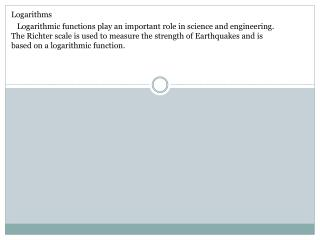DownloadDownload PresentationLogarithms

# Logarithms

Download Presentation## Logarithms

- - - - - - - - - - - - - - - - - - - - - - - - - - - E N D - - - - - - - - - - - - - - - - - - - - - - - - - - -
##### Presentation Transcript

1. Logarithms Logarithmic functions play an important role in science and engineering. The Richter scale is used to measure the strength of Earthquakes and is based on a logarithmic function.

2. Logarithms Logarithmic functions play an important role in science and engineering. The Richter scale is used to measure the strength of Earthquakes and is based on a logarithmic function. Logarithms are basically a restatement of known facts about exponents shown using special symbolism.

3. Logarithms Logarithmic functions play an important role in science and engineering. The Richter scale is used to measure the strength of Earthquakes and is based on a logarithmic function. Logarithms are basically a restatement of known facts about exponents shown using special symbolism.

4. Logarithms Logarithmic functions play an important role in science and engineering. The Richter scale is used to measure the strength of Earthquakes and is based on a logarithmic function. Logarithms are basically a restatement of known facts about exponents shown using special symbolism. Consider this rule :

5. Logarithms Logarithmic functions play an important role in science and engineering. The Richter scale is used to measure the strength of Earthquakes and is based on a logarithmic function. Logarithms are basically a restatement of known facts about exponents shown using special symbolism. Consider this rule : - where “b” is a base, raised to some exponent “u”

6. Logarithms Logarithmic functions play an important role in science and engineering. The Richter scale is used to measure the strength of Earthquakes and is based on a logarithmic function. Logarithms are basically a restatement of known facts about exponents shown using special symbolism. Consider this rule : - where “b” is a base, raised to some exponent “u” - What exponent “u” should I raise “b” to and get “v” ?

7. Logarithms Logarithmic functions play an important role in science and engineering. The Richter scale is used to measure the strength of Earthquakes and is based on a logarithmic function. Logarithms are basically a restatement of known facts about exponents shown using special symbolism. Let’s try some…

8. Logarithms Logarithmic functions play an important role in science and engineering. The Richter scale is used to measure the strength of Earthquakes and is based on a logarithmic function. Logarithms are basically a restatement of known facts about exponents shown using special symbolism. Let’s try some…

9. Logarithms Logarithmic functions play an important role in science and engineering. The Richter scale is used to measure the strength of Earthquakes and is based on a logarithmic function. Logarithms are basically a restatement of known facts about exponents shown using special symbolism. Let’s try some…

10. Logarithms Logarithmic functions play an important role in science and engineering. The Richter scale is used to measure the strength of Earthquakes and is based on a logarithmic function. Logarithms are basically a restatement of known facts about exponents shown using special symbolism. Let’s try some…

11. Logarithms Logarithmic functions play an important role in science and engineering. The Richter scale is used to measure the strength of Earthquakes and is based on a logarithmic function. Logarithms are basically a restatement of known facts about exponents shown using special symbolism. Let’s try some…

12. Logarithms Logarithmic functions play an important role in science and engineering. The Richter scale is used to measure the strength of Earthquakes and is based on a logarithmic function. Logarithms are basically a restatement of known facts about exponents shown using special symbolism. Let’s try some…

13. Logarithms Logarithmic functions play an important role in science and engineering. The Richter scale is used to measure the strength of Earthquakes and is based on a logarithmic function. Logarithms are basically a restatement of known facts about exponents shown using special symbolism. Let’s try some…

14. Logarithms Logarithmic functions play an important role in science and engineering. The Richter scale is used to measure the strength of Earthquakes and is based on a logarithmic function. Logarithms are basically a restatement of known facts about exponents shown using special symbolism. Let’s try some…

15. Logarithms Logarithm’s to the base 10 are called “common logarithms”.

16. Your calculator has a “LOG” button that solves ALL base 10 logarithms. Just plug in “b” and it will give “u”

17. A commonly used logarithm in real world applications such as compound interest is the Natural Logarithm.